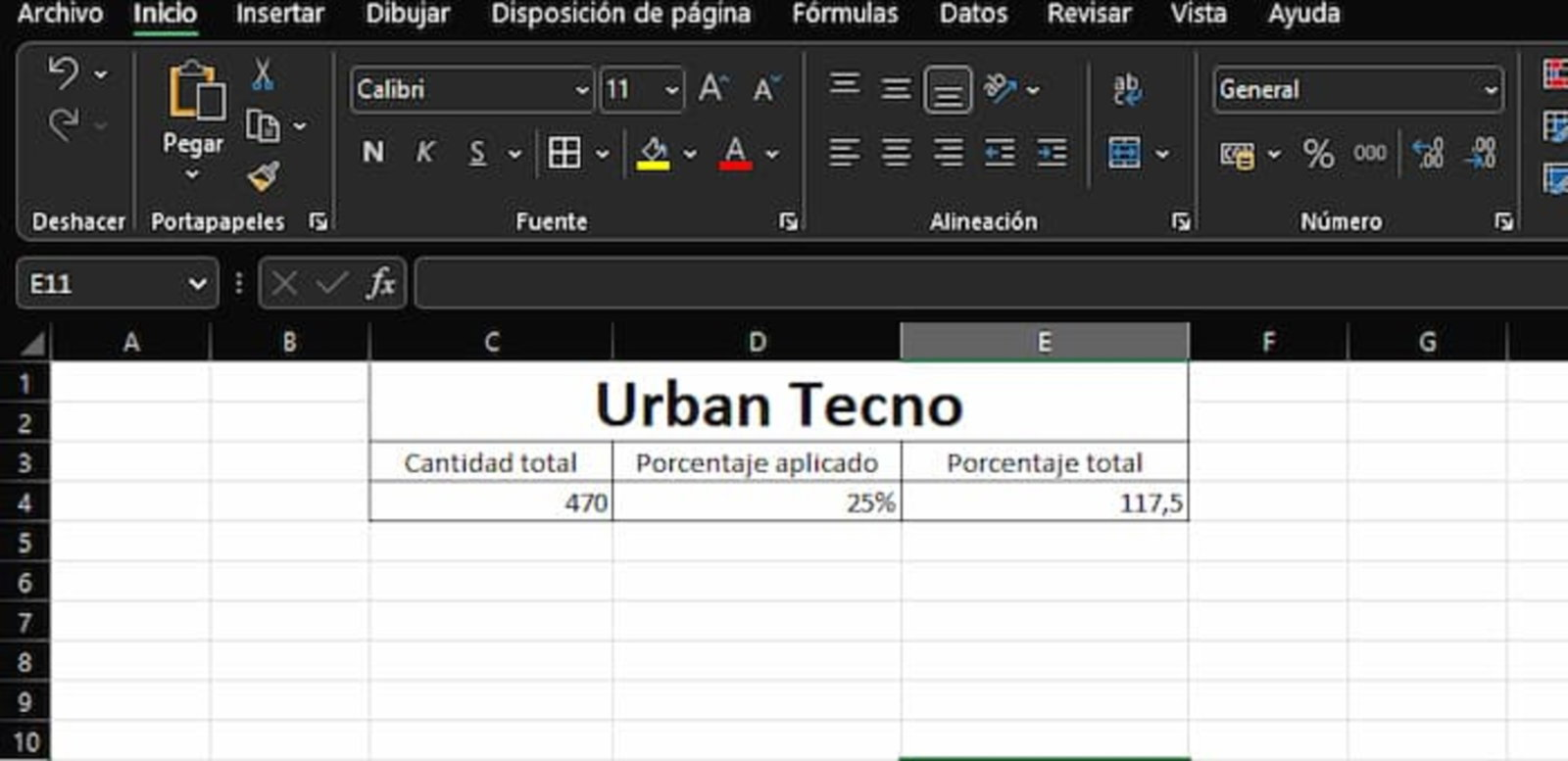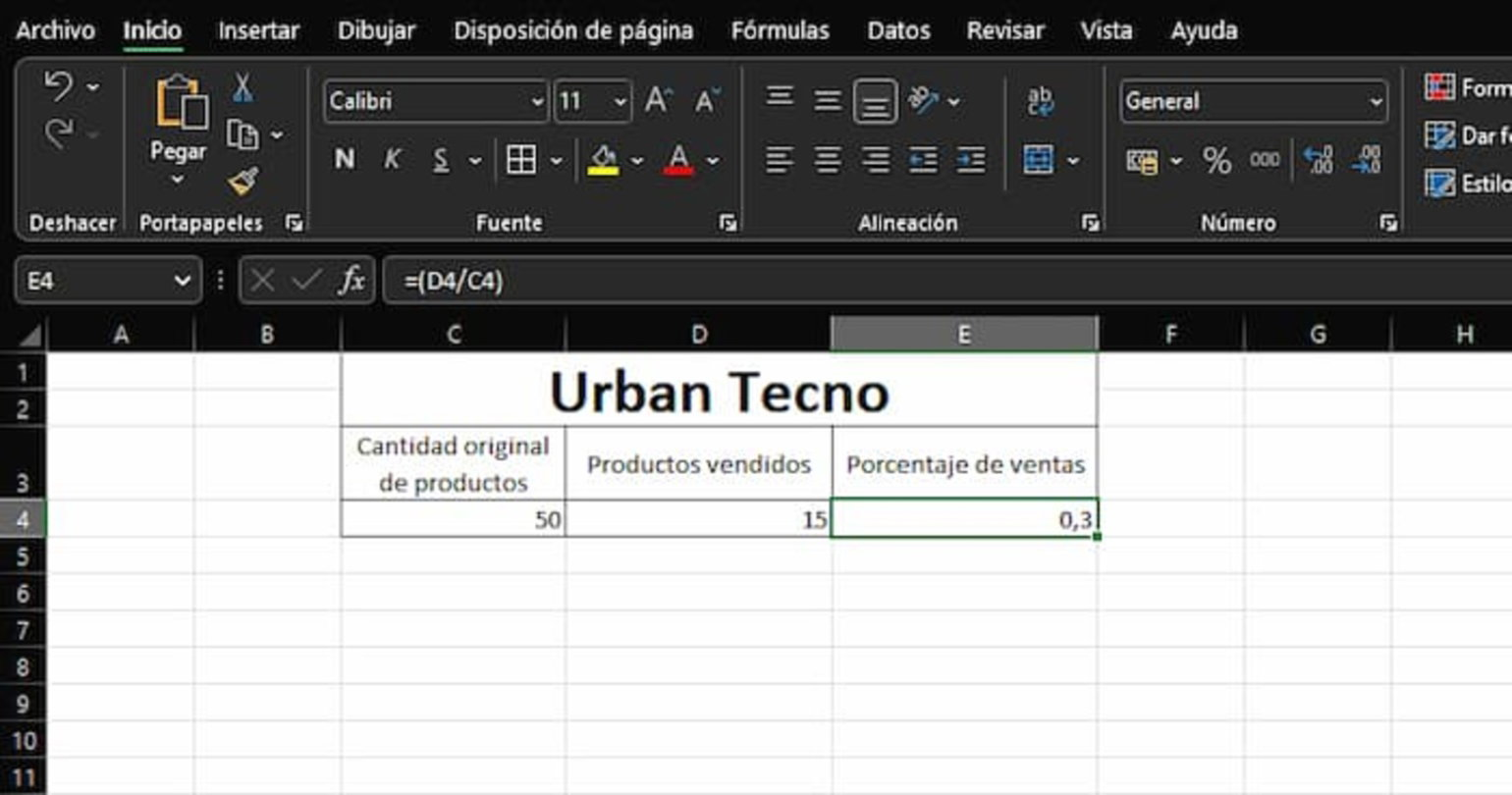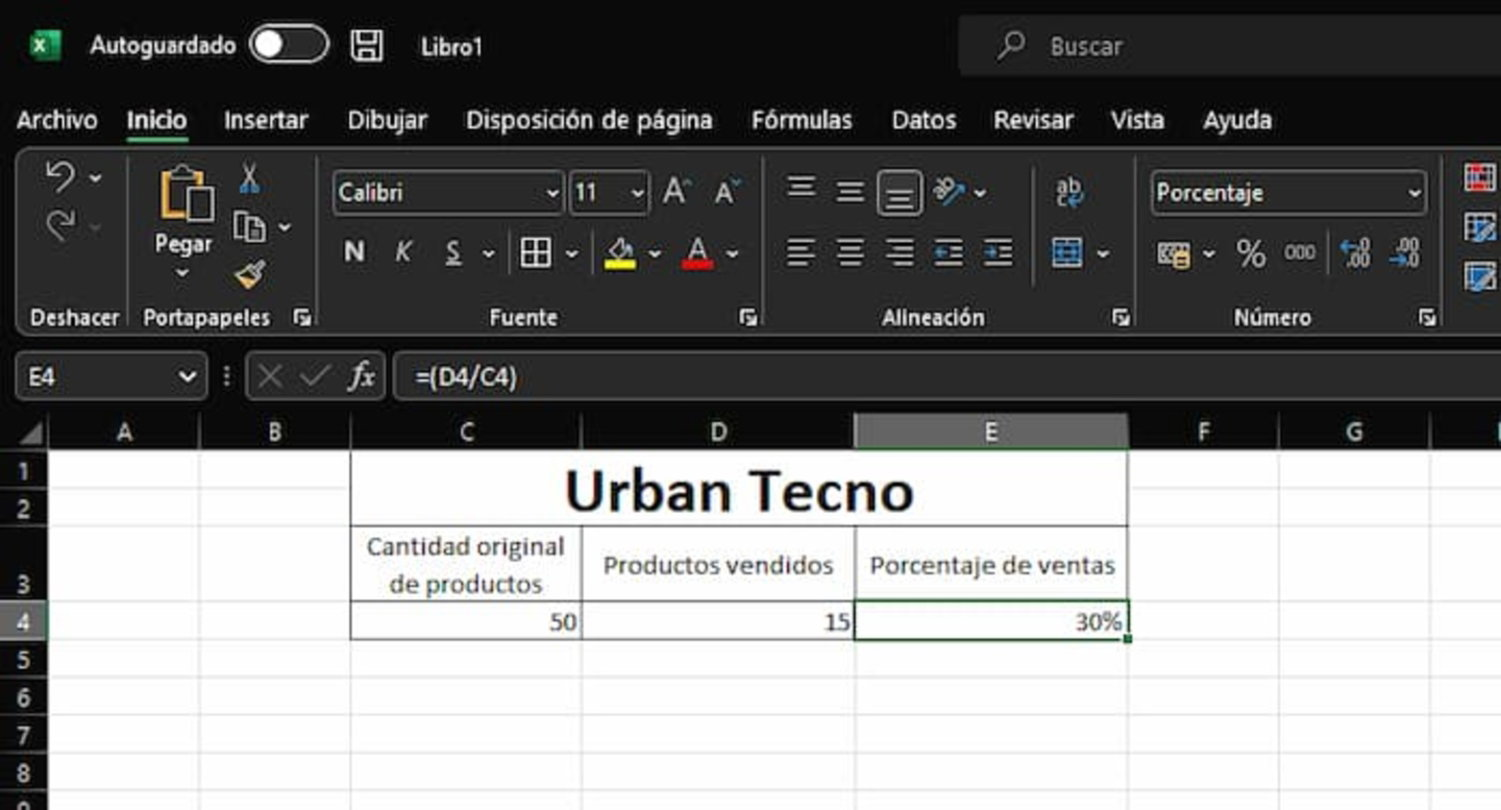Sunday, September 24, 2023
Latest:

### Tech Today Info

Technology Write For Us

Technology

# How to calculate a percentage in Excel

In Excel you have several ways to calculate a percentage quickly and easily.
One of the most important math operations to know when working with Excel, especially if you’re in charge of anything related to buying and selling , is how to calculate the percentage of an amount. Fortunately, Microsoft’s spreadsheet office tool allows you to do this easily , although you do need to know some very useful formulas and their functions .

Knowing how to work with Excel is very important and gives you useful tools at work . That is why it is recommended that you learn the basics, including some beginner formulas that can be very helpful. And one of the most interesting operations that you should know has to do with the calculation of percentages.

Therefore, in the next few lines we will tell you how you can perform this operation in a simple way , which will not take more than a couple of seconds.

• How to calculate the percentage of an amount in Excel
• Another formula to know the percentage in Excel
• How to convert a decimal number to a percentage

## How to calculate the percentage of an amount in Excel

To begin, we will start from the situation in which you are interested in knowing the percentage of a specific quantity. This can, for example, help you find out how much the actual discount is in some shopping deals and many other scenarios. And you just have to follow the steps that we will show you below.So you can calculate the percentage that is applied to an amount in a simple way and using Excel

• The first thing you should do is access Microsoft Excel from your computer and open a new workbook or spreadsheet.
• In one cell you must write the total amount you have and in the other you must enter the percentage that will be applied.
• Once you have the cells with their respective information, it will be time to enter the formula.
• In a third cell, you must enter the formula: =(total amount * percentage to apply) and press Enter .
• In this way, the mathematical operation will be carried out.
Read More:   What Can I Do With a Degree in English?

In our example, we have written the total amount in cell “C4”, while in cell “D4” we have entered the percentage to be applied . Following the formula that we have told you about, it would look something like this: in cell “F4” write =(C4*D4) and press Enter .

After this, the mathematical operation will be carried out, giving as a result that 25% of 470 is 117.5. This can be very useful to know how much money you could save if, for example, you see that a product that originally costs 470 euros has a 25% discount.

## Another formula to know the percentage in Excel

On the other hand, it can also happen that what you need is to discover the percentage of a quantity to have a more complete perspective . In this case, the formula that is used is slightly different from the previous one, but, in the same way, it is very simple.If, on the contrary, you want to know how much is the percentage of something, then you must resort to this formula

In the event that, for example, you have a certain number of products and you have sold some and you want to know how much the percentage of total sales is , then you can use this formula in your Excel sheet.

• The first thing you should do is access your spreadsheet in Excel.
• Then, you must position yourself in a cell and write the total amount that you originally had.
• While, in the other cell, you must write the number of products you have sold, according to our example.
• After this, in the next cell you will have to write the formula: =(number of products sold / total number of products) .
• If you press Enter , the mathematical operation will be carried out, which will give you a result.
Read More:   Windows 10 Tips and Tricks

Following the example we have mentioned, if you originally had 50 products, which you have entered in cell “C4”, and of this total you have sold 15, which you have registered in cell “D4” , you probably want to know what percentage these correspond to. sales. So, you just have to plug the amounts into the formula and you will have a result.

Thus, the formula would be =(D4/C4) , which returns 0.3 . This is a decimal number, but one that is necessary to know the percentage.

### How to convert a decimal number to a percentageLastly, you need to change the cell format to Percentage to change the decimal number

Now that the division operation has resulted in a decimal number, you’re closer to knowing the percentage of total sales , as it only takes one last step.

You must click on the cell that contains the result of 0.3 and then click on the “%” icon found in the Home tab of Excel and in the Number section . After this, the amount will automatically become 30%.

Calculating the percentage of an amount is very simple, because you only have to know the values ​​and the formula that you will have to apply.#### Rose

Rose is a technology enthusiast and a writer. She had the interest to write articles related to technology, software, Mobiles, Gadgets and many more.